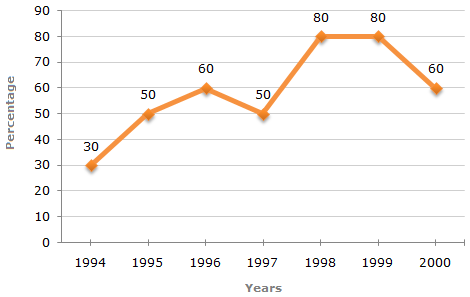# Data Interpretation - Line Charts - Discussion

### Discussion :: Line Charts - Line Chart 5 (Q.No.3)

The following line graph gives the percentage of the number of candidates who qualified an examination out of the total number of candidates who appeared for the examination over a period of seven years from 1994 to 2000.

Percentage of Candidates Qualified to Appeared in an Examination Over the Years3.

If the number of candidates qualified in 1998 was 21200, what was the number of candidates appeared in 1998?

 [A]. 32000 [B]. 28500 [C]. 26500 [D]. 25000

Explanation:

The number of candidates appeared in 1998 be x.

 Then, 80% of x = 21200x = 21200 x 100 = 26500 (required number). 80